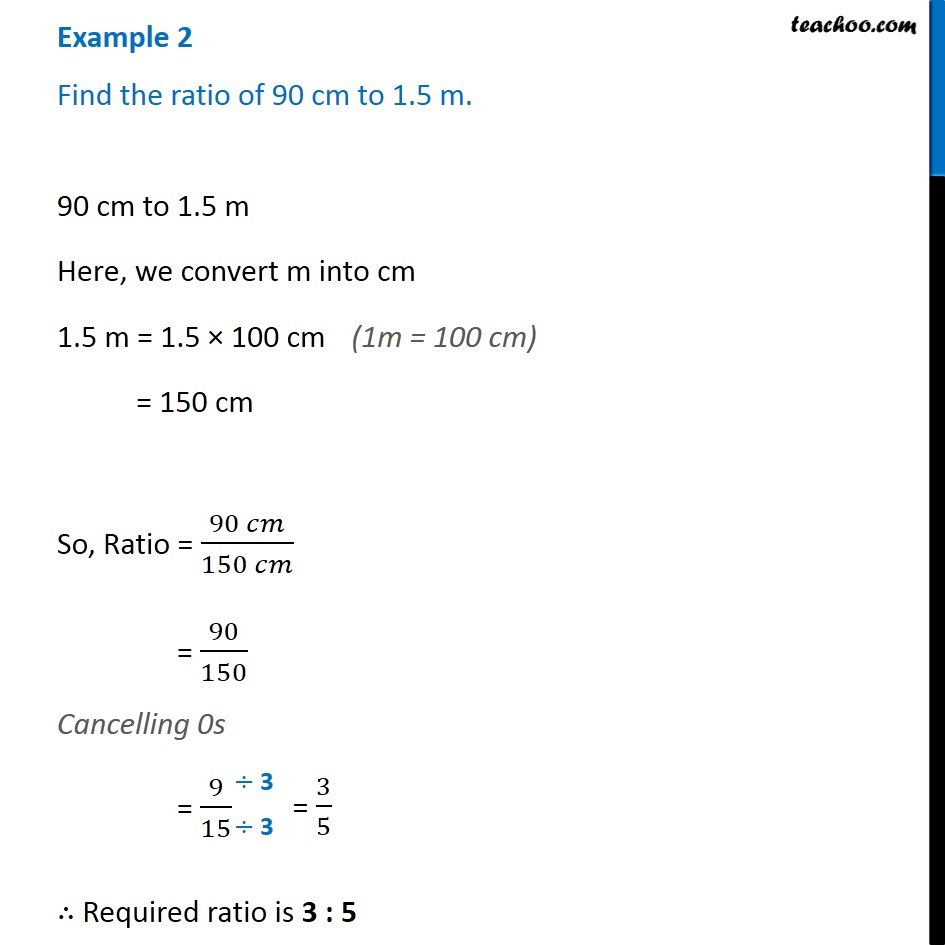Finding ratio by changing units

Chapter 12 Class 6 Ratio And Proportion
Concept wiseIntroducing your new favourite teacher - Teachoo Black, at only ₹83 per month

### Transcript

Example 2 Find the ratio of 90 cm to 1.5 m. 90 cm to 1.5 m Here, we convert m into cm 1.5 m = 1.5 × 100 cm = 150 cm So, Ratio = (90 𝑐𝑚)/(150 𝑐𝑚) = 90/150 Cancelling 0s = 9/15 ∴ Required ratio is 3 : 5